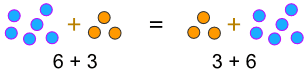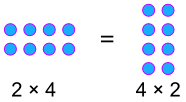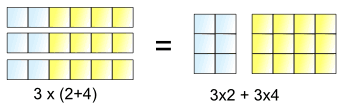# Commutative, Associative and Distributive Laws

Wow! What a mouthful of words! But the ideas are simple.

## Commutative Laws

The "Commutative Laws" say we can swap numbers over and still get the same answer ...

a + b  =  b + a

### Example:... or when we multiply:

a × b  =  b × a

### Example:### Commutative Percentages!

Because a × b  =  b × a it is also true that a% of b  =  b% of a

### Example: 8% of 50 = 50% of 8, which is 4Why "commutative" ... ?

Because the numbers can travel back and forth like a commuter.

## Associative Laws

The "Associative Laws" say that it doesn't matter how we group the numbers (i.e. which we calculate first) ...

(a + b) + c  =  a + (b + c)... or when we multiply:

(a × b) × c  =  a × (b × c)

### Examples:

 This: (2 + 4) + 5  =  6 + 5  =  11 Has the same answer as this: 2 + (4 + 5)  =  2 + 9  =  11

 This: (3 × 4) × 5  =  12 × 5  =  60 Has the same answer as this: 3 × (4 × 5)  =  3 × 20  =  60

### Uses:

Sometimes it is easier to add or multiply in a different order:

### What is 19 + 36 + 4?

19 + 36 + 4  =  19 + (36 + 4)
=  19 + 40 = 59

Or to rearrange a little:

### What is 2 × 16 × 5?

2 × 16 × 5  =  (2 × 5) × 16
=  10
× 16 = 160

## Distributive Law

The "Distributive Law" is the BEST one of all, but needs careful attention.

This is what it lets us do:3 lots of (2+4) is the same as 3 lots of 2 plus 3 lots of 4

So, the can be "distributed" across the 2+4, into 3×2 and 3×4

And we write it like this:

a × (b + c)  =  a × b  +  a × c

Try the calculations yourself:

• 3 × (2 + 4)  =  3 × 6  =  18
• 3×2 + 3×4  =  6 + 12  =  18

Either way gets the same answer.

In English we can say:

We get the same answer when we:

• multiply a number by a group of numbers added together, or
• do each multiply separately then add them

### Uses:

Sometimes it is easier to break up a difficult multiplication:

### Example: What is 6 × 204 ?

6 × 204  =  6×200 + 6×4
=  1,200 + 24
=  1,224

Or to combine:

### Example: What is 16 × 6 + 16 × 4?

16 × 6 + 16 × 4  =  16 × (6+4)
= 16 × 10
=  160

We can use it in subtraction too:

### Example: 26×3 - 24×3

26×3 - 24×3 = (26 - 24) × 3
=  2 × 3
=  6

We could use it for a long list of additions, too:

### Example: 6×7 + 2×7 + 3×7 + 5×7 + 4×7

6×7 + 2×7 + 3×7 + 5×7 + 4×7
= (6+2+3+5+4) × 7
= 20 × 7
= 140

## . . . but don't go too far!

The Commutative Law does not work for subtraction or division:

### Example:

• 12 / 3 = 4, but
• 3 / 12 = ¼

The Associative Law does not work for subtraction or division:

### Example:

• (9 – 4) – 3 = 5 – 3 = 2, but
• 9 – (4 – 3) = 9 – 1 = 8

The Distributive Law does not work for division:

### Example:

• 24 / (4 + 8) = 24 / 12 = 2, but
• 24 / 4 + 24 / 8 = 6 + 3 = 9

## Summary

 Commutative Laws: a + b  =  b + a a × b  =  b × a Associative Laws: (a + b) + c  =  a + (b + c) (a × b) × c  =  a × (b × c) Distributive Law: a × (b + c)  =  a × b  +  a × c